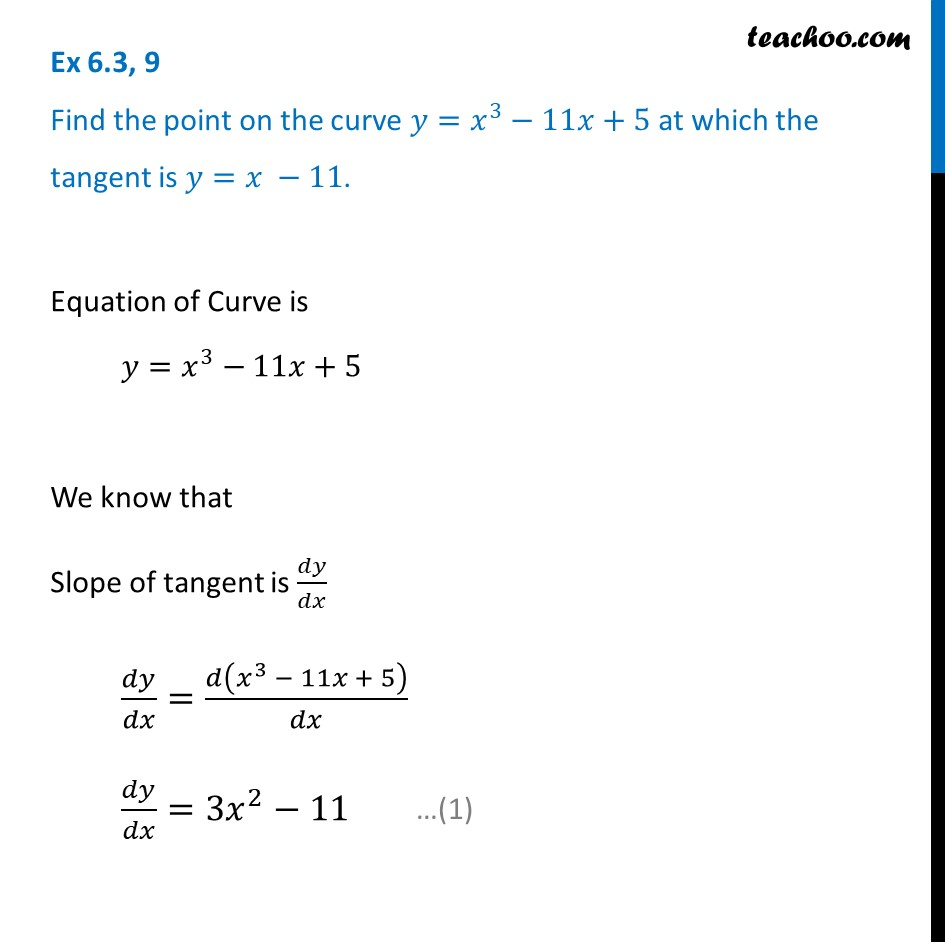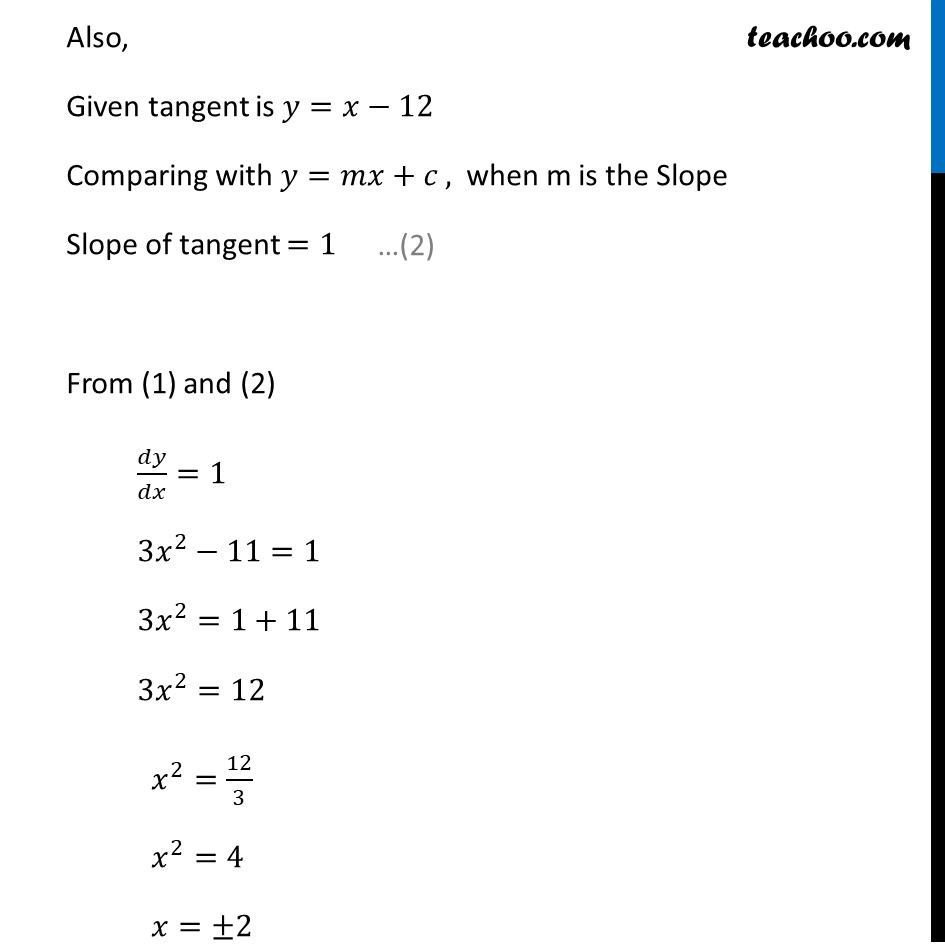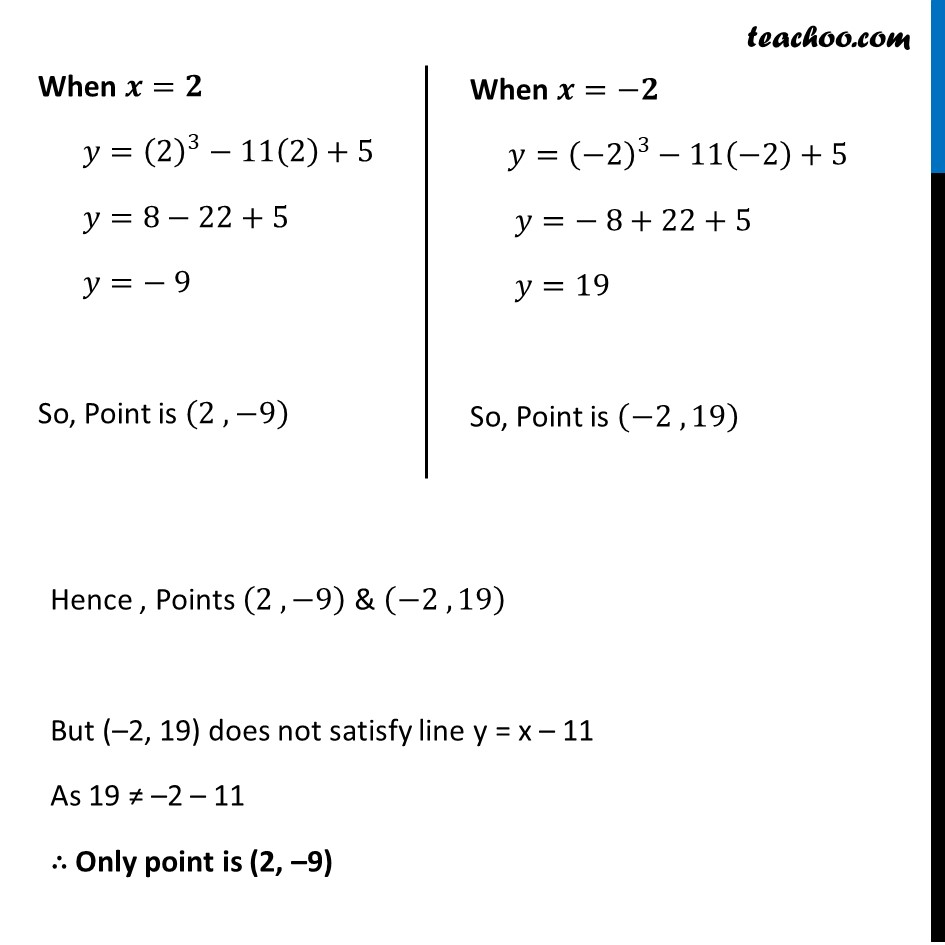Finding point when tangent is parallel/ perpendicular

Chapter 6 Class 12 Application of Derivatives
Concept wiseIntroducing your new favourite teacher - Teachoo Black, at only ₹83 per month

### Transcript

Ex 6.3, 9 Find the point on the curve 𝑦=𝑥^3−11𝑥+5 at which the tangent is 𝑦=𝑥 −11.Equation of Curve is 𝑦=𝑥^3−11𝑥+5 We know that Slope of tangent is 𝑑𝑦/𝑑𝑥 𝑑𝑦/𝑑𝑥=𝑑(𝑥^3 − 11𝑥 + 5)/𝑑𝑥 𝑑𝑦/𝑑𝑥=〖3𝑥〗^2−11 Also, Given tangent is 𝑦=𝑥−12 Comparing with 𝑦=𝑚𝑥+𝑐 , when m is the Slope Slope of tangent =1 From (1) and (2) 𝑑𝑦/𝑑𝑥=1 3𝑥^2−11=1 3𝑥^2=1+11 3𝑥^2=12 𝑥^2=12/3 𝑥^2=4 𝑥=±2 When 𝒙=𝟐 𝑦=(2)^3−11(2)+5 𝑦=8−22+5 𝑦=− 9 So, Point is (2 , −9) When 𝒙=−𝟐 𝑦=(−2)^3−11(−2)+5 𝑦=− 8+22+5 𝑦=19 So, Point is (−2 , 19) Hence , Points (2 , −9) & (−2 , 19) But (–2, 19) does not satisfy line y = x – 11 As 19 ≠ –2 – 11 ∴ Only point is (2, –9)